# Number System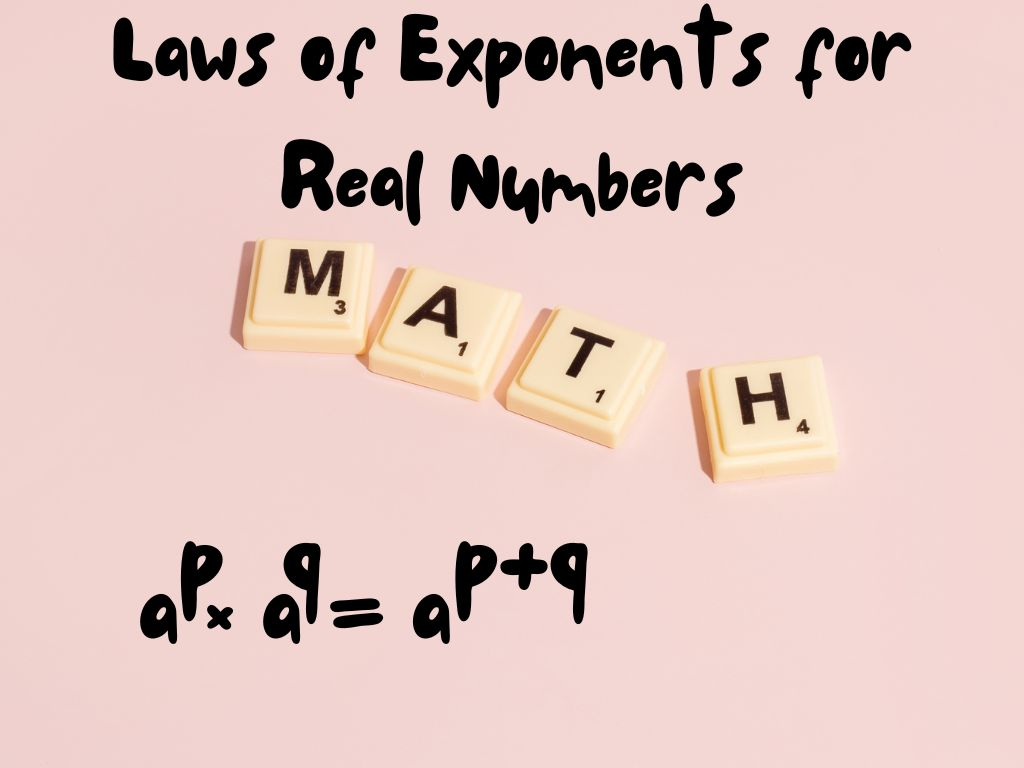## Laws of Exponents for Real Numbers Class 9th

When we multiply a number continuously by itself till a certain number, then exponents are used to write these numbers in a suitable and convenient form. In mathematics, we use different laws of exponents to solve large calculations. In this section, we will study different laws of exponents for real numbers. Before understanding the laws …## Operations on Real Numbers Class 9th

In this section, we shall study different mathematical operations on real numbers such as addition, subtraction, multiplication, and division. We know that rational numbers and irrational numbers are real numbers so we’ll apply operations on them. For Rational Numbers As we have learnt in previous classes, rational numbers satisfy the commutative, associative, and distributive properties …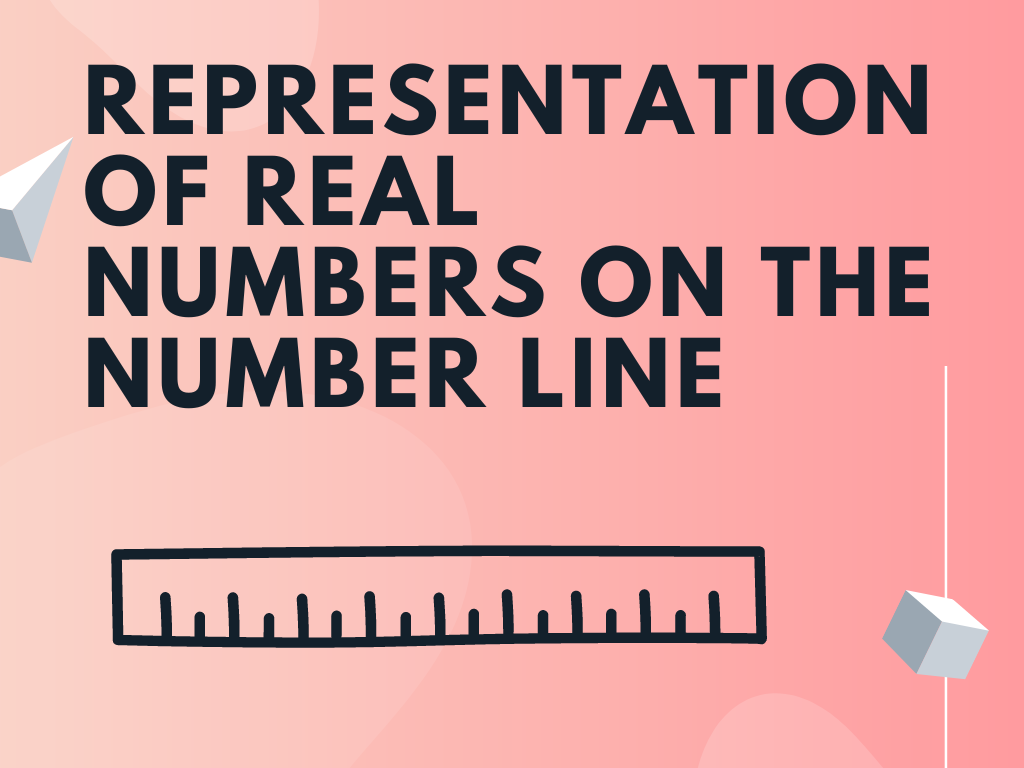## Representation of Real Numbers on the Number Line Class 9th

As per the knowledge of the previous classes, we are familiar with the representation of different types of numbers on the number line like the representation of natural numbers, whole numbers, integers, and rational numbers. In this section, we’ll learn the representation of real numbers on the number line including irrational numbers also. We’ll represent …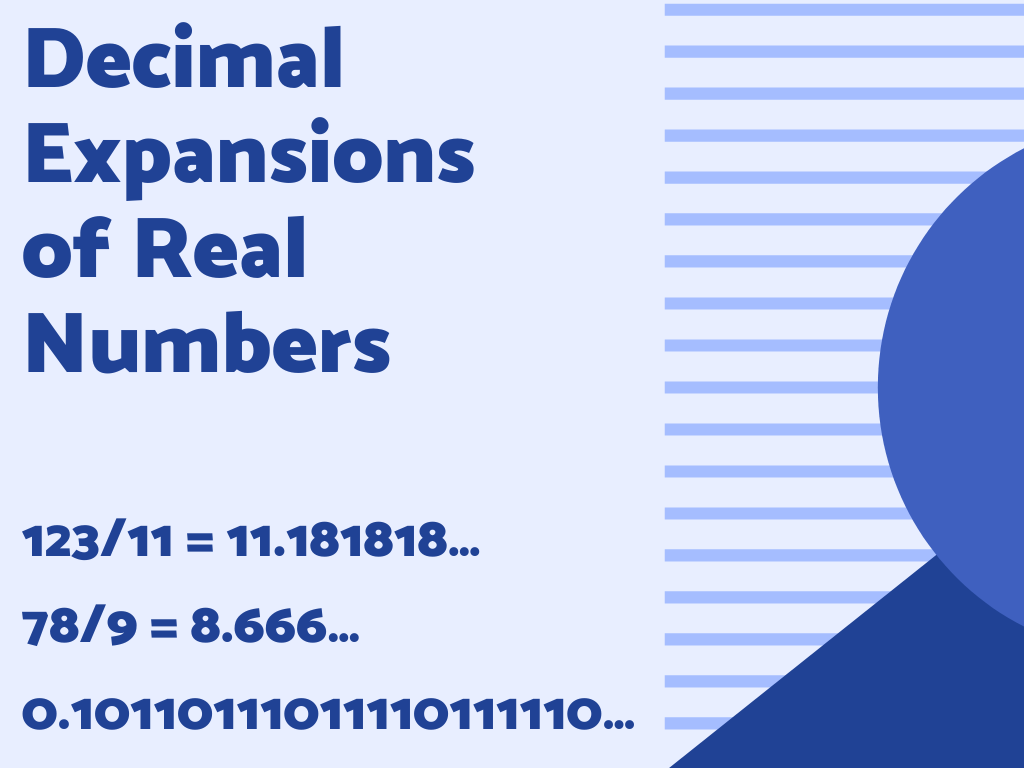## Decimal Expansions of Real Numbers Class 9th

As we know that collections of all rational numbers and irrational numbers are known as real numbers. In this section, we shall study decimal expansions of real numbers means decimal expansions of rational numbers and irrational numbers. First, we start with rational numbers. Decimal Expansions of Rational Numbers Rational numbers are in the form of …## Powers and Exponents Class 8th

Introduction In the previous class, we have already studied powers and exponents. We know that exponents are used to write large numbers in a suitable and convenient form. In large calculations, powers and exponents are very important and useful. In class 8th, we shall explore more about powers and exponents with the help of their …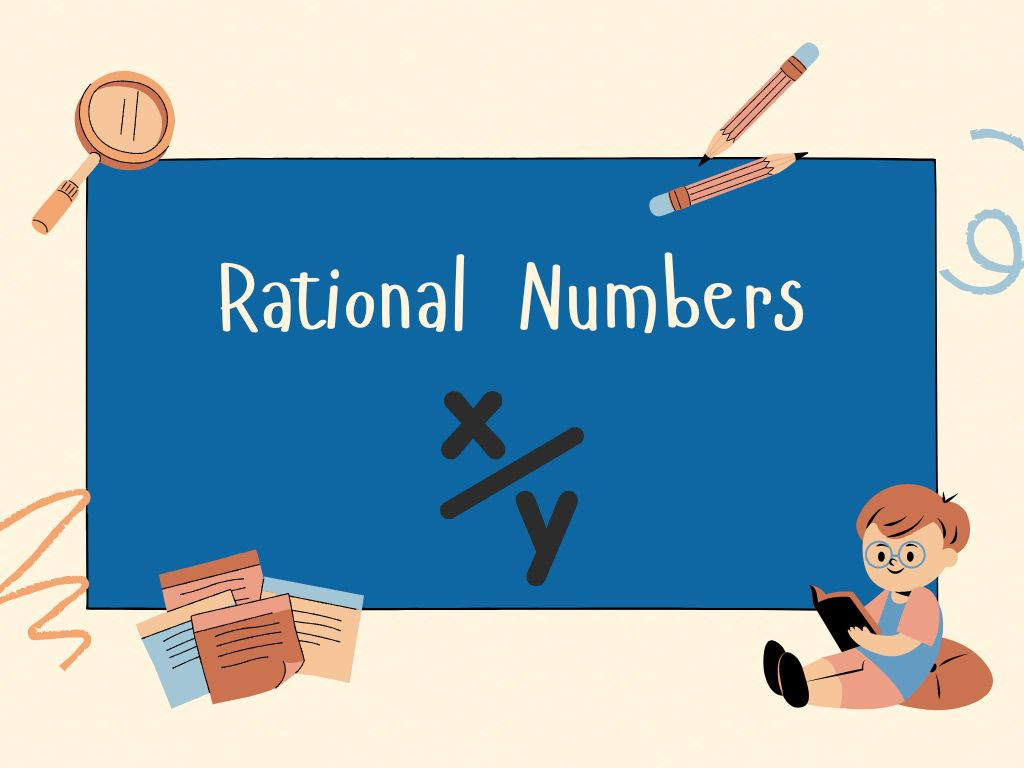## Rational Numbers Class 8th

Introduction In the number system, we have already studied whole numbers, natural numbers, integers, fractions, decimal numbers, etc. All of these types of numbers have different properties. In class 8th, we shall study Rational numbers, their definition and properties, the representation of rational numbers on the number lines, etc. Definition of Rational Number A number …## Real Numbers Class 8th

Introduction As the name suggests, these numbers are real for mathematical calculations. There are different types of real numbers. In class 8th, we will get into the definition, types with examples, and properties of Real Numbers. Definition All rational numbers and irrational numbers are called Real Numbers. Real numbers are denoted by R. Examples – …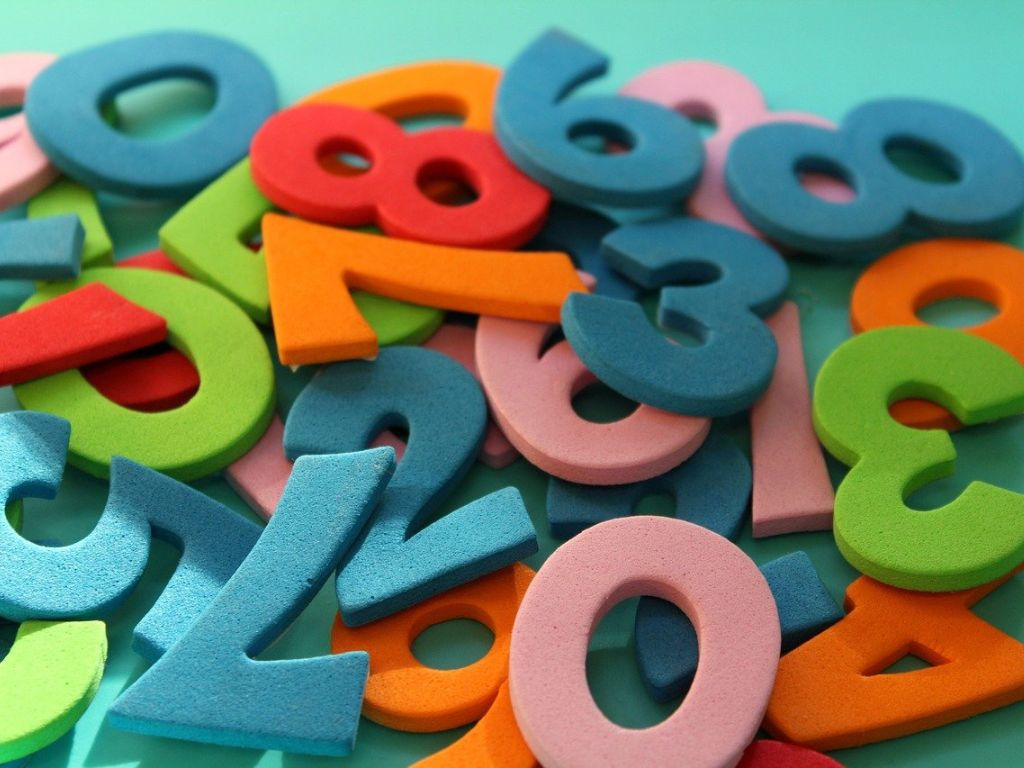## Whole Numbers Class 6th

Introduction We know that counting numbers begin with 1, 2, 3, 4, 5……… these numbers are called natural numbers. It means all the counting numbers are called Natural numbers. Natural numbers come under the whole numbers. In class 6th, whole numbers are as follows. Definition All the counting numbers including zero (0) are called Whole …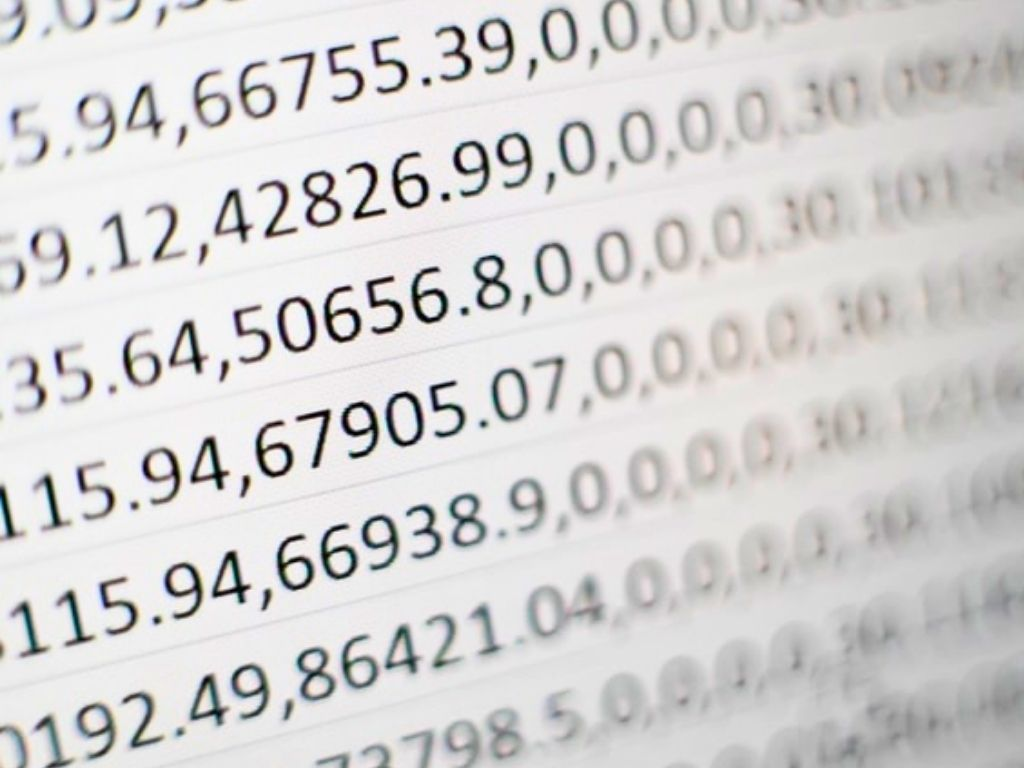## Decimal Expansion of Rational Numbers Class 10th

Introduction We have already studied that the number which can be written in the form of p/q is called a Rational Number. Where p and q are integers and q ≠ 0. The decimal expansion of rational numbers is either a terminating decimal expansion or a non-terminating repeating (recurring) decimal expansion. In this section, we …